×

Search anything:

# Association in Unsupervised Learning

#### Machine Learning (ML) Deep LearningGet this book -> Problems on Array: For Interviews and Competitive Programming

## What is association?

In Data Mining, association refers to the process where co occurrences and dependencies between different features in a dataset are discovered. The discovered patterns can then help in understanding the underlying structure of the data and provides insights into the same. In simple words, association refers to the phenomenon where certain entities are always found together because they are related in some way, and the aim is to extract those relations and form insights on the same and utilizing said insights to fulfill the needed requirements.

### Association vs Causality

Often times, association is confused with causality. This should not be done as they are two related but distinct concepts. Association refers to a relationship between two variables that tend to occur together, but it does not necessarily imply a cause-and-effect relationship between them while causality refers to a relationship between two variables where one variable directly affects the other variable. For example, if there is a positive association between the sales of bread and that of butter, it does not necessarily mean that the sales of bread ‘cause’ the sales of butter or vice versa. It merely means that they co-occur, that’s all.

Below listed are some applications of association.

One of the most common applications of association in unsupervised learning is in market basket analysis. Market basket analysis is used to identify which items are frequently purchased together by customers. This information can be used to improve product placement and increase sales. For example, if customers frequently purchase bread and milk together, the store can place them in close proximity to each other to encourage more sales.

Anomaly Detection

Another common application of association in unsupervised learning is in anomaly detection. Anomaly detection involves identifying data points that are significantly different from the rest of the data. This technique can be used to detect fraudulent transactions, identify potential equipment failures, and diagnose medical conditions.

Healthcare

In the healthcare industry, association analysis is used for identifying the relationships between different symptoms and diseases. This information can be used for early diagnosis and treatment of diseases.

Social Sciences

In the social sciences, association analysis is used for identifying the relationships between different variables. For example, in psychology, association analysis can be used to identify the relationship between different personality traits.

## Algorithms for Association Rule Mining

For the purposes of this article, we shall be focusing on unsupervised learning approaches to implement association in Python, examples being Apriori Algorithm and FP Growth Algorithm. For those unfamiliar with Unsupervised learning, it is a type of machine learning that enables the discovery of patterns and relationships in data without being provided with any explicit supervision or labels as opposed to supervised learning techniques where data points are labeled.

### Apriori Algorithm

Given by R. Agrawal and R. Srikant in 1994, the apriori algorithm is a classic algorithm for association rule mining. It is based on the idea of frequent itemset generation. The algorithm starts by identifying the frequent item sets and then generates the association rules based on these frequent item sets.

### Concepts Used-

Support- Fraction of transactions that contain a particular itemset. Support of item I is defined as the number of transactions containing I divided by the total number of transactions.
Confidence - Confidence is the likelihood that item Y is also bought if item X is bought,  It’s calculated as the number of transactions containing X and Y divided by the number of transactions containing X.
Frequent itemsets - This term refers to all the itemsets whose support satisfies the minimum support threshold.

The Apriori Algorithm works by generating a list of all possible itemsets and then pruning the list to only those that are frequent. To determine the frequent itemsets, support and confidence values are used as metrics.
It then generates candidate itemsets of increasing size iteratively, and then prunes them according to their support. The algorithm first detects each individual item with a support value higher than a predetermined threshold. The algorithm combines the common itemsets from the previous iteration to produce candidate itemsets in following iterations. Only the frequent itemsets are kept for the following iteration after these candidate itemsets have been trimmed depending on their support.

When no additional frequent item sets can be located, the algorithm repeats this process. The patterns that commonly appear in the dataset are represented by the frequent itemsets that are produced.

Time and space complexity -
The Apriori algorithm is considered expensive both in terms of computation and memory, as it requires multiple passes over the dataset and generates a large number of candidate itemsets. In the worst case, the time complexity may be exponential, but this can be avoided by the use of various optimization techniques. Since this algorithm also requires the storing of candidate itemsets and their support counts, as well as the frequent itemsets found in each iteration, it requires a significant amount of memory as well. Overall, the time and space complexity of the Apriori algorithm can be a limiting factor for very large datasets, but this can be overcome through the use of various optimization techniques.

Implementation in Python -

We shall be using jupyter notebooks an open source software for this task.

Dataset Used - Groceries Dataset

``````import numpy as np
import pandas as pd

``````

The above code imports the prerequisite libraries and data, while displaying the first 5 rows of the data.

Output-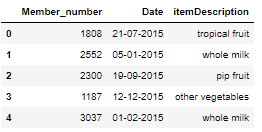Checking data types and quality -

``````data.isnull().sum() #checks for null values
data.info() #describes the dataset
data.shape #shows length and width of the dataset
``````

We shall be now installing the apyori library from where we can import the apriori function.

``````!pip install apyori #this command installs the apyori library
from apyori import apriori #import apriori
``````

Before proceeding forward with rule mining, we need to transform (or rather, encode) the data into a format readable by the algorithm, which basically is the market basket of each customer. An example would be the table shown below

The first step would be the conversion of data into a sparse matrix, where the presence of 1 signifies the presence of a product in a basket.
``````products = data['itemDescription'].unique()
dummy = pd.get_dummies(data['itemDescription'])
data.drop(['itemDescription'], inplace =True, axis=1)
data = data.join(dummy)
``````

The above code snippet transforms the dataset into a sparse matrix, suitable for further use. It now looks like this.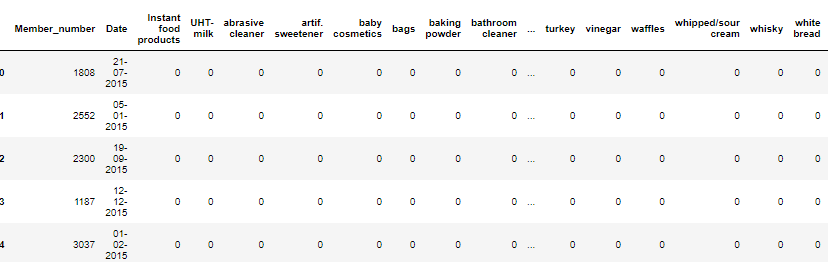The second step shall be the replacement of the 1's in the table with the name of the corresponding product.

``````def productnames(x):
for product in products:
if x[product] >0:
x[product] = product
return x

data1 = data1.apply(productnames, axis=1)
``````

Output-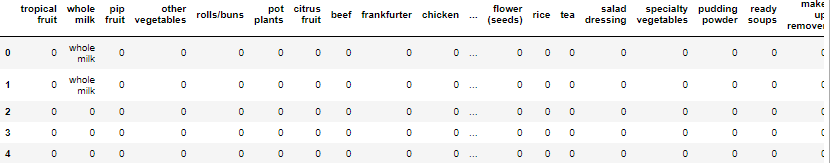The final step in preprocessing the data would be generating the market basket of each customer.

``````x = data1.values
x = [sub[~(sub==0)].tolist() for sub in x if sub [sub != 0].tolist()]
transactions = x
transactions[0:10]
``````

These are the first ten market baskets as generated by the code.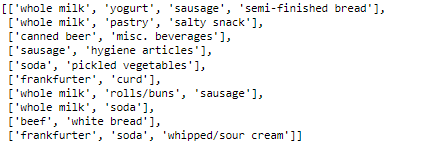Now we shall be applying the apriori algorithm and then generate association rules from the data. We shall be taking minimum support as 0.0003 and minimum confidence as 0.05.

``````rules = apriori(transactions, min_support = 0.00030, min_confidence = 0.05, min_lift = 3, max_length = 2, target = "rules")
association_results = list(rules)
for item in association_results:

pair = item
items = [x for x in pair]

print("Rule : ", items, " -> " + items)
print("Support : ", str(item))
print("Confidence : ",str(item))
print("Lift : ", str(item))

print("--------------------------------")
``````

The following association rules are generated by this code.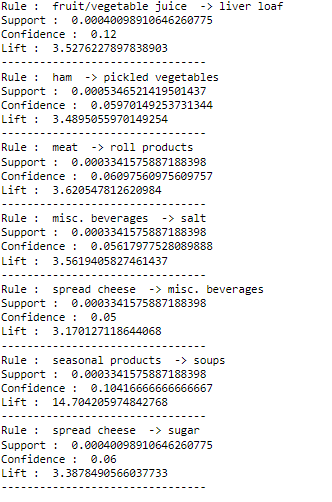This is an end product of the apriori algorithm and insights can be generated from the derived rules.

### FP Growth Algorithm

While we were going through the working of the apriori algorithm, we observed that it was expensive in terms of memory and computation. It was also observed to be not suited for large datasets due to the need to generate candidate itemsets. These limitations often make the implementation of Apriori algorithm difficult.

Proposed by J.Han, The FP (Frequent Pattern) growth algorithm is an alternative method to discover association rules. This algorithm generates an FP-tree - a compressed representation of the transaction database, after performing a single scan of the dataset. The FP-tree is built by sequentially adding each transaction to the tree while keeping track of the frequency of each item. For the purpose of streamlining the tree structure and minimising its size, the items are added in descending order of frequency.

After generating the tree, the algorithm then searches it for frequent itemsets, or groups of items, which happen to be present in a predetermined number of transactions. The technique locates collections of items that have the same prefix path in the tree by starting at the bottom and working its way up. For each frequent item, the algorithm creates a conditional pattern base, which is a collection of transactions containing both the frequent item and all of the items in its prefix route. Once all common itemsets have been identified, the algorithm repeats the process on the conditional pattern base.

The technique efficiently explores the FP-tree by pruning branches that do not lead to frequent itemsets using a depth-first search approach. Due to this, it can effectively mine frequent itemsets from big datasets containing a variety of unique items.

### Running time of FP Growth Algorithm

The algorithm requires only two iterations over the dataset to construct the FP-tree and mine frequent itemsets, which makes it more efficient than the Apriori Algorithm in many cases.

In terms of space complexity, the FP-growth algorithm is also relatively efficient, especially for datasets with a large number of items and a low minimum support threshold. The algorithm constructs a compact data structure called the FP-tree, which can be stored in memory more efficiently than the candidate itemsets generated by Apriori. The space complexity of the FP-growth algorithm is O(n*m), where n is the number of transactions and m is the number of distinct items in the dataset.

### Comparing FP Growth Algorithm and Apriori Algorithm-

• The FP-growth algorithm is often faster than the Apriori approach since it typically requires fewer operations and consumes less memory.
• In comparison to the candidate itemsets produced by Apriori, the FP-growth algorithm generates a compact data structure (the FP-tree) that can be stored in memory more effectively.
• As  it does not require multiple passes over the data, the FP-growth method can handle huge datasets and high-dimensional data better than Apriori.
• In some circumstances, Apriori may outperform FP-growth, particularly in the case of dense datasets or datasets with very low minimum support criteria.
• In certain cases, it may be more cumbersome to draw an FP tree, if done manually when compared to Apriori.

### Implementing FP Growth Algorithm in Python

We shall be using the transaction data we obtained earlier and continue from there. But before moving forward, we need to install the required modules first.

``````!pip install pyfpgrowth
import pyfpgrowth
``````

This snippet shall install pyfpgrowth in your machine and import it.

Having a look at the transaction data-

``````transactions[0:10]
``````Now we shall be identifying frequent patterns from the data.

``````patterns = pyfpgrowth.find_frequent_patterns(transactions, 10)
patterns
sorted_patterns = {key: value for key, value in sorted(patterns.items(), key=lambda item: item,reverse=True)}

sorted_patterns
``````

The above snippet returns a large dictionary, having it's first 5 terms as-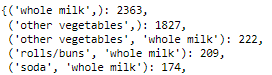Finally, we shall be generating the association rules. 0.02 is taken to be the minimum cutoff.

``````rules = pyfpgrowth.generate_association_rules(patterns, 0.02)
rules
``````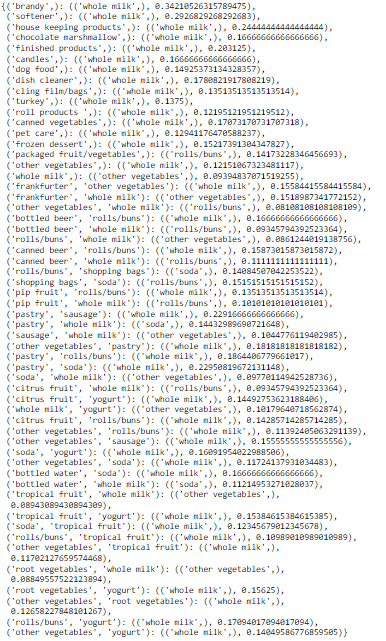## Conclusion

Association is a powerful tool for finding patterns and correlations in data in unsupervised learning. It aids in finding the interesting connections or relationships between various elements in a big dataset. Applications for association rules include retail, healthcare, and the social sciences. We have covered the definition of association, applications of association, unsupervised learning algorithms used for this, and how to put those algorithms into practice in this article. Before moving on, let's have a look at a few questions.

## Question 1

#### Does association necessarily imply causation?

Yes
No
Sometimes
Can't Say
Association refers to a relationship between two variables that tend to occur together, but it does not necessarily imply a cause-and-effect relationship between them.

## Question 2

#### Is FP Growth Algorithm always a superior choice compared to Apriori lgorithm?

Yes
No
Although FP Growth is often seen to be more efficient than Apriori, there are cases when the Apriori algorithm is better suited to handle the data. E.g. in the case of dense datasets or datasets with very low minimum support criteria.

With this article at OpenGenus, you must have the complete idea of Association in Unsupervised Learning.#### Ambarish Deb

Final Year Computer Science Student at Sri Guru Gobind Singh College Of Commerce; ML Intern at OpenGenus.

Association in Unsupervised Learning